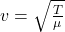## A stretched string is 2.11 m long and has a mass of 19.5 g. When the string oscillates at 440 Hz , which is the frequency of the standard A

Question

A stretched string is 2.11 m long and has a mass of 19.5 g. When the string oscillates at 440 Hz , which is the frequency of the standard A pitch, transverse waves with a wavelength of 15.3 cm travel along the string. Calculate the tension T in the string.

in progress 0
2 months 2021-07-31T13:02:15+00:00 1 Answers 5 views 0

The tension of the string is 41.876 N

Explanation:

Given;

length of the string, L = 2.11 m

mass of the string, m = 19.5 g = 0.0195 kg

frequency of the wave, f = 440 Hz

wavelength, λ = 15.3 cm = 0.153 m

The velocity of the wave is given by;

v = fλ

v = 440 x 0.153

v = 67.32 m/s

Also the velocity of the wave is given bywhere;

μ is mass per unit length = 0.0195 / 2.11 = 0.00924 kg/m

T is the tension of the string

T = v²μ

T = (67.32)²(0.00924)

T = 41.876 N

Therefore, the tension of the string is 41.876 N